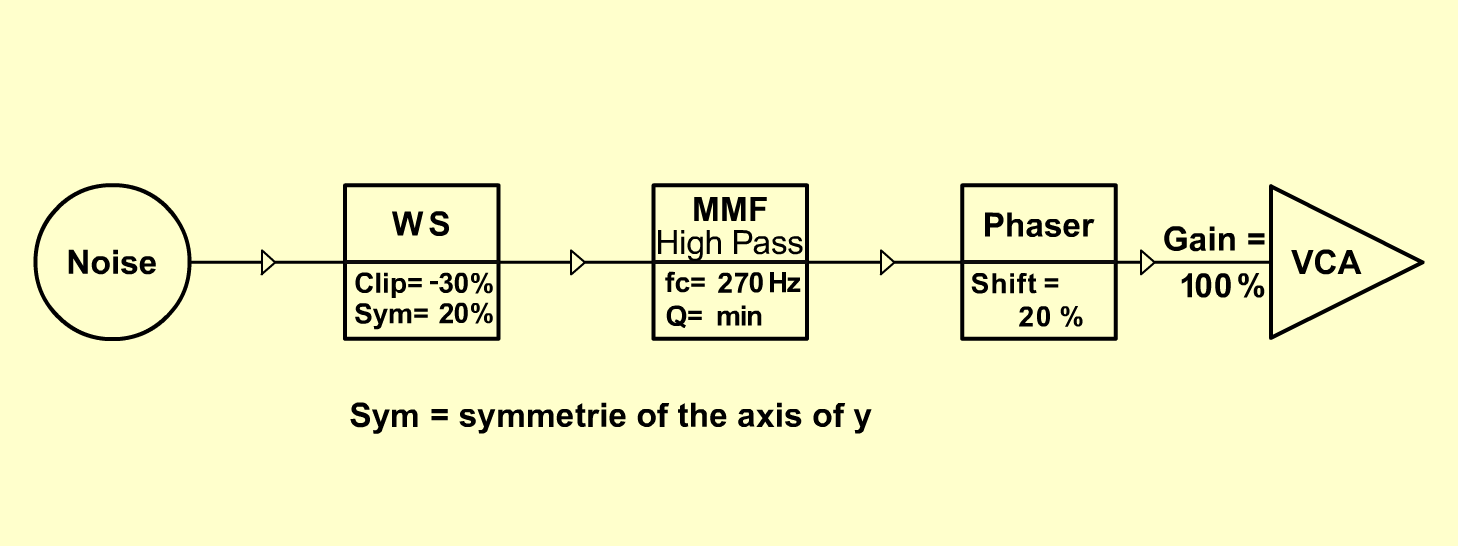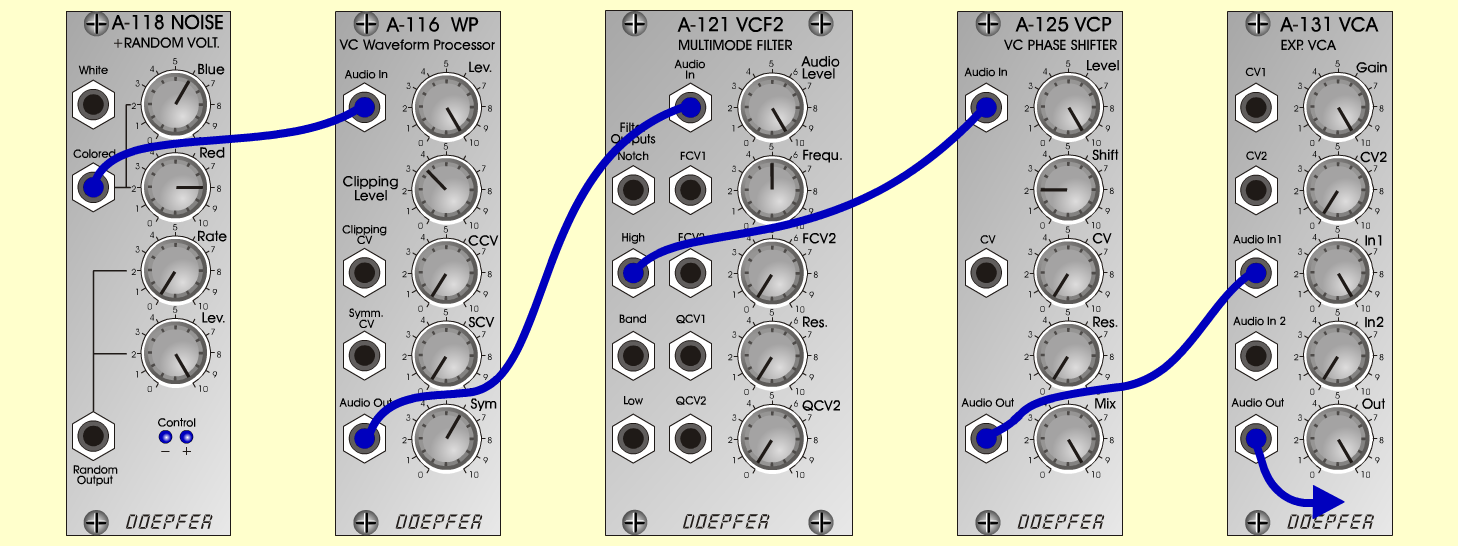R a i nN a t u r eD o e p f e r A - 100 Connections: Settings: A-118 (Colored)  <=>  A-116 (Audio In) A-116 (Audio Out)  <=>  A-121 (Audio In) A-121 (High)  <=>  A-125 (Audio In) A-125 (Audio Out)  <=>  A-131 (Audio In) A-131 (Audio Out)  <=> Amplifier A-118 (Blue = 6, Red = 8, Rate = 0, Level = 10) A-116 (Lev = 10, Clip Level = 3.5, Sym = 6) A-121 (Audio Level = 10, Freq = 5, Res = 0) A-125 (Level = 10, Shift = 2, Res = 0, Mix = 10) A-131 (Gain = 10, Audio In 1 = 10,  Audio Out = 10) Josef MuellerSound samples Bad weather (with Stormy & Thunder) Rain